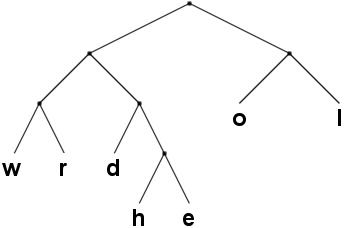### Problem Statement

Plugin users: There is a picture in the problem statement.

A coding tree is a proper binary tree (in which every node has either zero or two children) where each leaf has a distinct character assigned to it. This allows us to encode a string made up of the characters in the leaves as follows: For every character in the string, find the path from the root of the coding tree to the matching leaf. If following the left child of a node, append a 0 to the encoding, otherwise a 1. For instance, the string "helloworld" would, using the tree below, be encoded as "011001111111100001000111010" (zeros are the left branches). To decode a string of 0's and 1's, one just reverses this procedure: start at the root of the tree and follow the edges downwards: 0 for left, 1 for right. When a leaf is reached, append the leaf's character to the output string, and continue from the root.One way (albeit not a very good one) to represent the coding tree is to describe for each character the path to the leaf. For instance, the tree in the picture above would be described as

```{"h 0110",
"e 0111",
"l 11",
"o 10",
"w 000",
"r 001",
"d 010"}
```

In this problem, however, some characters in the binary codes in this representation have been damaged (and replaced by a question mark, '?'). Given a damaged representation and an encoded message, determine if it's possible to uniquely decode the encoded message, using the properties above about a well formed coding tree (see example 0). You may also use the fact that the encoded message must be fully decodable (see example 3). You are guaranteed that there will be at least one way to decode the message.

Create a class DamagedTree containing the method decode which takes a String[], tree, representing a damaged representation of a coding tree in the format above, and a String[], encodedMessage, the encoded message (concatenate the elements in encodedMessage to get the full encoded message). If there is a way to uniquely decode this message, return this decoded message. Otherwise return the empty string (see example 1).

### Definition

 Class: DamagedTree Method: decode Parameters: String[], String[] Returns: String Method signature: String decode(String[] tree, String[] encodedMessage) (be sure your method is public)

### Notes

-A '?' in tree must be replaced with either '0' or '1'.

### Constraints

-At most 15 characters in tree will be a '?'.
-tree will contain between 2 and 50 elements, inclusive.
-Each element in tree will contain between 3 and 50 characters inclusive.
-The first character in the elements of tree will be 'A'-'Z', 'a'-'z' or a space.
-The second character in the elements of tree will be a space.
-The remaining characters in the elements of tree will be either '0', '1' or '?'.
-The first characters in the elements of tree will be distinct.
-There will be at least one way to replace the '?' in tree so that tree describes a valid coding tree that can fully decode encodedMessage.
-encodedMessage will contain between 1 and 50 elements, inclusive.
-Each element in encodedMessage will contain between 1 and 50 characters, inclusive.
-encodedMessage will only contain the characters '0' and '1'.

### Examples

0)

 ```{"h 01??", "e 0111", "l ??", "o 10", "w 000", "r 001", "d 010"}``` `{"011001111111100001000111010"}`
`Returns: "helloworld"`
 For the letter 'l' we can deduce that the first '?' must be a '1'. It can't be a '0' because both the codes "00" and "01" wouldn't be valid codes since they are prefixes of other existing codes. Hence it's a '1', and then the second '?' for 'l' must also be '1' so it doesn't conflict with the code for the letter 'o'. The first '?' in 'h' can't be a '0' since the code for 'd' would then be a prefix to the code for 'h'. Hence it's a '1' and the last '?' in 'h' must be a '0'. The complete definition then becomes like the one in the problem statement, and there is of course only one way to decode the encoded message.
1)

 ```{"h 01??", "e 011?", "l ??", "o 10", "w 000", "r 001", "d 010"}``` `{"011001111111100001000111010"}`
`Returns: ""`
 Same as above, except that the last character in 'e' has been replaced with a '?'. Now it's no longer possible to tell which of 'h' and 'e' should be "0110" and "0111", and the message could be decoded both as "helloworld" and "ehlloworld". Hence the method returns "".
2)

 ```{"h 01??", "e 011?", "l ??", "o 10", "w 000", "r 001", "d 010"}``` `{"000100","0111010"}`
`Returns: "world"`
 tree is the same as above, but to decode the message we don't need to know if 'h' or 'e' is "0110" or "0111".
3)

 ```{"A ??", "B ???", "C ???", "D 1"} ``` `{"0000"}`
`Returns: "AA"`
 The only way to decode the message is if 'A' is "00" ('B' and 'C' would be "010" and "011", in some order). Note that 'B' or 'C' can't be "000" because then we would only partially be able to decode the message. We are allowed to assume that the encoded message should be possible to completely decode.
4)

 ```{"a 0????", "b 0????", "c 0???", "d 0??", "e 0?", " ?"}``` ```{"1111111111111111111111111", "1111111111111111111111111"}```
`Returns: "                                                  "`
 The return value is a string containing 50 spaces.

#### Problem url:

http://www.topcoder.com/stat?c=problem_statement&pm=3040

#### Problem stats url:

http://www.topcoder.com/tc?module=ProblemDetail&rd=5883&pm=3040

Yarin

#### Testers:

PabloGilberto , lbackstrom , vorthys

Brute Force